『風水夏夏叫學堂』居家環境風水篇
\\動畫X脫口秀X超實用生活風水\\

『風水夏夏叫學堂』

「居家建築風水課程」預計於2016/8月上線
「商用建築風水課程」

6

39分鐘

• 緒論
• 1 風水概要試看 04:13
• 選房建地之風水
• 2 山脈試看 06:12
• 3 水源試看 06:46
• 房屋周圍之風水
• 4 道路試看 07:53
• 5 周遭建築物試看 05:38
• 房屋外型
• 6 房相試看 08:39

### 開始分享你的學習成果吧！

<%if list.length == 0 %><%else%> <%props list%>

<%if prop.type == 'image' %>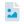圖片檔 <%else prop.type == 'pdf' %>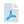PDF <%else prop.type == 'youtube' %>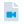Youtube 影片連結 <%else prop.type == 'ppt' %>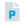PowerPoint <%else prop.type == 'sound' %>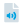錄音檔 <%/if %>

<%:prop.title%>

<%/props%> <%/if%>

### 作業觀摩<%props data%>

<%if prop.type == 'image' %><%else prop.type == 'pdf' %><%else prop.type == 'youtube' %><%else prop.type == 'ppt' %><%else prop.type == 'sound' %><%/if %> 作業<%>~numToZH(~toInt(key) + 1)%> <%:prop.title%>

<%:~loadTmpl('homework-card', prop.rows)%>
<%/props%>
<%props data%>
<%if prop.type == 'image'%> <%if prop.cover %>
<%:~isGif(prop.cover)%>
<%else%>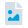<%/if%> <%else prop.type == 'pdf' %>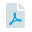<%else prop.type == 'youtube' %>
<%else prop.type == 'ppt' %>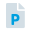<%else prop.type == 'sound' %>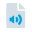<%/if%>
<%if prop.visible == 1 %> <%/if %>

<%:~formatDate(prop.create, 'YYYY-MM-DD')%>

<%:prop.title%>

<%if prop.isAuthor == true %> 刪除 編輯 <%/if %>

<%:prop.comment_count%>

<%/props%>

# 來關注最新的課程資訊吧！<%props data%>

<%:~formatDate(prop.publishDate, 'YYYY/MM/DD')%>

<%:prop.title%>

<%if ~root.isStudent === false && ~root.isTeacher === false && prop.permission === 'private' %>

<%else%> <%:prop.content%> <%/if%>
<%/props%>